• 一种 clamp 操作，然后将一定范围内的intensity线性拉伸到某个指定区间。 记某像素点的为p，对各像素点做以下操作： f(p)={ap<aMp−ab−aa≤p≤bMp>bf(p)= \begin{cases} a& {p < a}\\ M\dfrac{p - a...
目录1 计算原理2 代码实现3 效果展示4 源码下载
1 计算原理
一种 clamp 操作，然后将一定范围内的intensity线性拉伸到某个指定区间。
记某像素点的值为p，对各像素点做以下操作：
$f(p)= \begin{cases} a& {p < a}\\ M\dfrac{p - a}{b - a} & {a \leq p \leq b}\\ M& {p > b} \end{cases}$
则将图像window在 $[a, b]$ 内，最大intensity为 $M$ .
2 代码实现
%% reading in the image
orgIMG = rgb2gray(imread("lena.jpg"));
figure(1);
subplot(1,2,1);
imshow(orgIMG);
title("Original IMG");
subplot(1,2,2);
imhist(orgIMG);
axis([0 255 0 800]);
title("The Histogram of the original img");

%% Doing intensity windowing
% Define parameters
a_min = 20;    % a_min <- 0~a_max
a_max = 220;    % a_max <- a_min~255
M = 220;        % intensity

orgIMG = double(orgIMG);

smaller = find(orgIMG <= a_min);
larger = find(orgIMG >= a_max);
middle = find(orgIMG > a_min & orgIMG < a_max);

orgIMG(smaller) = a_min;
orgIMG(larger) = M;
orgIMG(middle) = M.*(orgIMG(middle)-a_min)./(a_max-a_min);

orgIMG = uint8(orgIMG);

%% Draw the new img
figure(2);
subplot(1,2,1);
imshow(orgIMG);
title("Modified IMG");
subplot(1,2,2);
imhist(orgIMG);
axis([0 255 0 800]);
title("The Histogram of the Modified img");


3 效果展示
原图：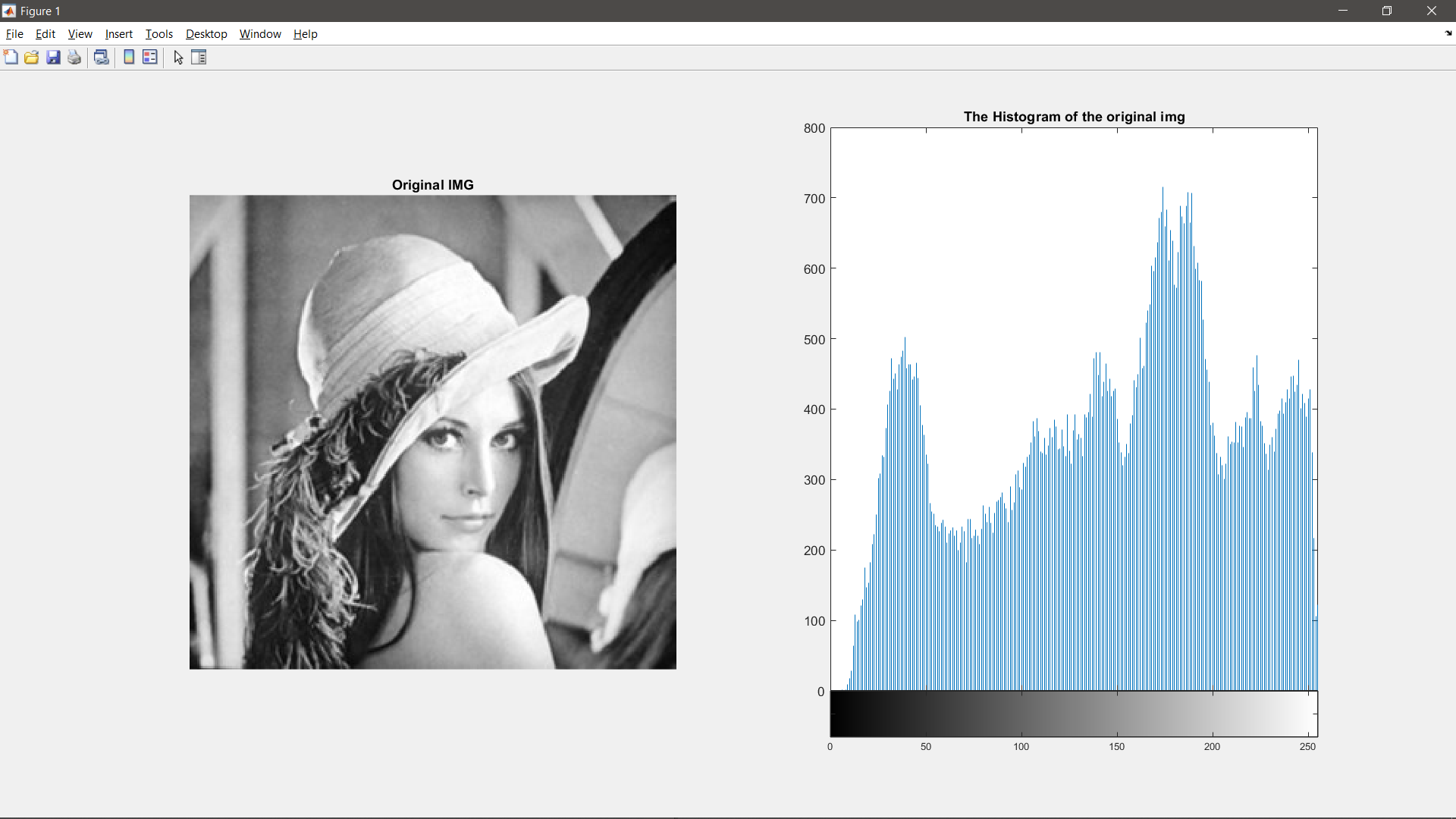$[a, b] = [20, 220], M = 220:$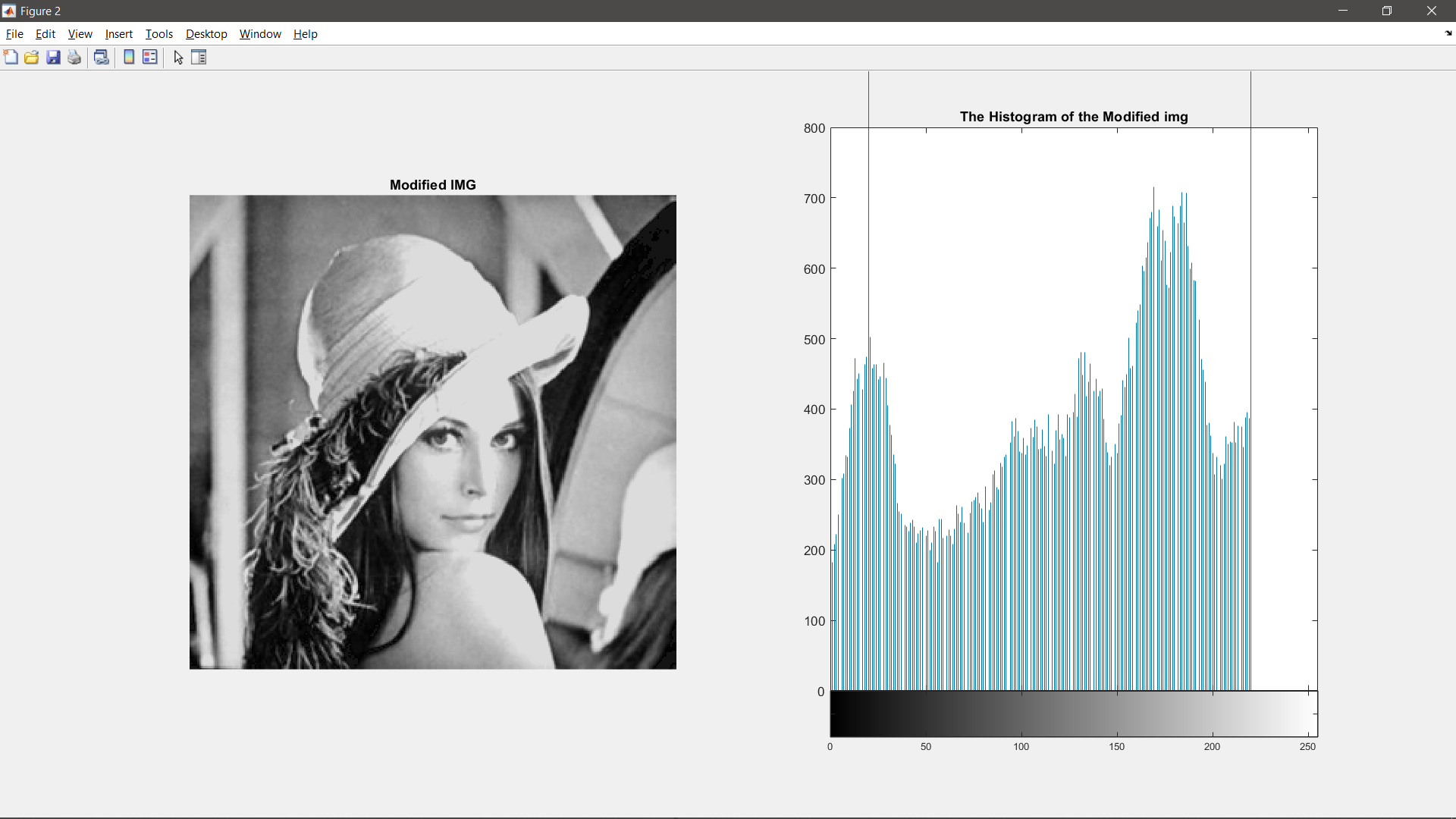4 源码下载
源码下载地址：点击此处


展开全文• 它将输入图像映射为输出图像，输出图像每个像素点的灰度值仅有对应的输入像素点的灰度值决定，运算结果不会改变图像内像素点之间的空间关系。其运算的数学关系式如下：其中，A（x，y）表示原图像，B（x，y）表示经过...
点运算又称为对比度增强、对比度拉伸或灰度变换，是一种通过图像中的每一个像素值进行运算的图像处理方式。。它将输入图像映射为输出图像，输出图像每个像素点的灰度值仅有对应的输入像素点的灰度值决定，运算结果不会改变图像内像素点之间的空间关系。其运算的数学关系式如下：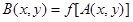其中，A（x，y）表示原图像，B（x，y）表示经过点运算处理后的图像，f表示点运算的关系函数。按照灰度变换的数学关系，点运算可以分为线性灰度变换、分段线性灰度变换和非线性灰度变换3种。接下来我们分别举例实现。1. 线性灰度变换假定原图像A（x，y）的灰度变换范围为[a，b]，处理后的图像B（x，y）的灰度变换范围为[c，d]，线性灰度变换运算的数学表达式为：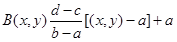在MATLAB图像处理工具箱中提供了一个灰度线性变换函数imadjust（）close all;clear all;clc;
%通过函数imadjust()对图像进行线性灰度变换
gamma=0.5;  %设定调整线性度取值
I=imread('F:/pao1.jpg');
R=I;  %将图像数据赋值给R
R(:,:,2)=0;  %将原图像变成单色图像，保留红色
R(:,:,3)=0;
R1=imadjust(R,[0.5 0.8],[0 1],gamma);  %利用函数imajust（）调整R的灰度，结果返回R1
G=I;
G(:,:,1)=0;  %将原图像变成单色图像，保留绿色
G(:,:,3)=0;
G1=imadjust(G,[0 0.3],[0 1],gamma);  %利用函数imajust（）调整G的灰度，结果返回G1
B=I;
B(:,:,1)=0;  %将原图像变成单色图像，保留蓝色
B(:,:,2)=0;
B1=imadjust(B,[0 0.3],[0 1],gamma);  %利用函数imajust（）调整B的灰度，结果返回B1
I1=R1+G1+B1;  %求变换后的RGB图像
figure,
subplot(131),imshow(I);
subplot(132),imshow(R);
subplot(133),imshow(R1);
figure,
subplot(131),imshow(I);
subplot(132),imshow(G);
subplot(133),imshow(G1);
figure,
subplot(131),imshow(I);
subplot(132),imshow(B);
subplot(133),imshow(B1);
figure,
subplot(121),imshow(I);
subplot(122),imshow(I1);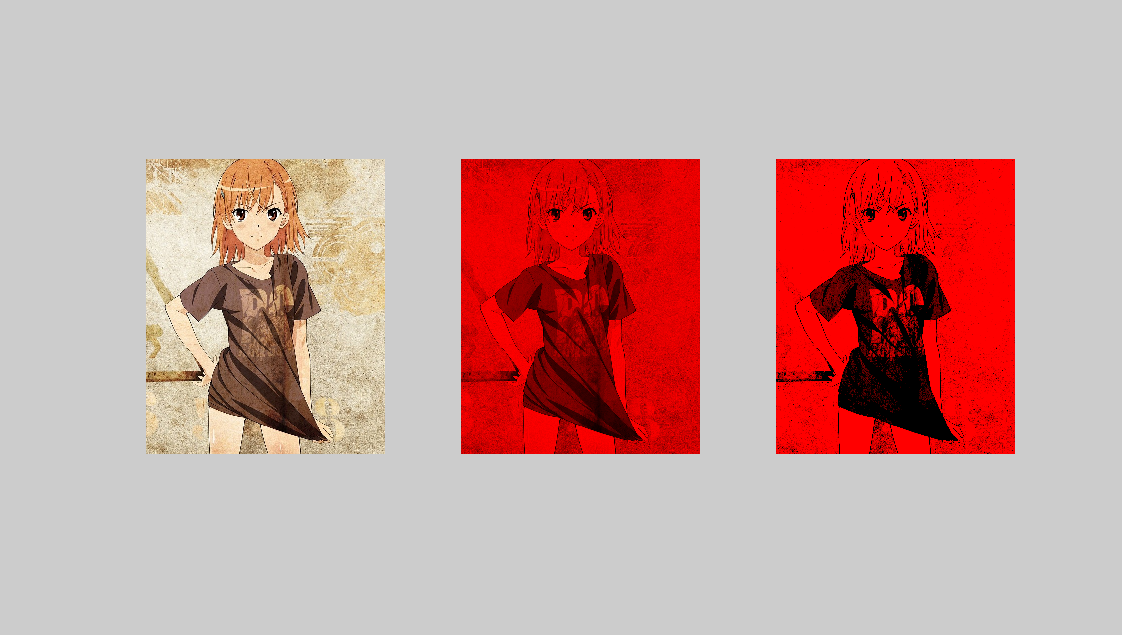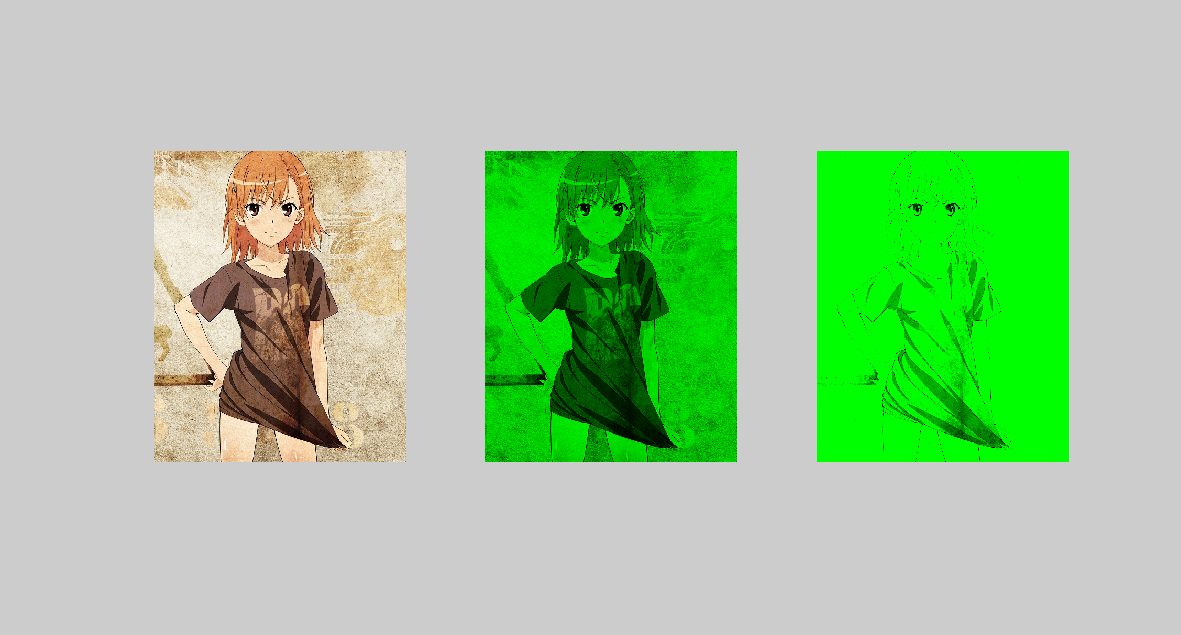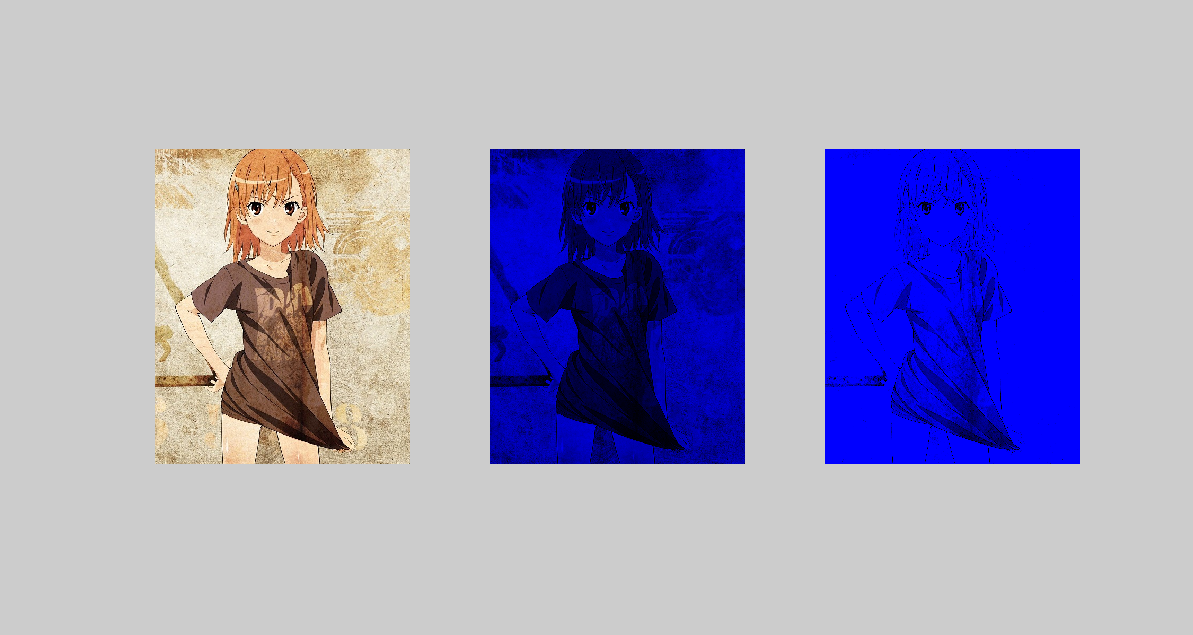2. 分段线性灰度变换为了突出图像中感兴趣的目标或者灰度区间，可采用分段线性法，将需要的图像细节灰度拉伸，对比度增强。3段线性变换法运算的数学表达式如下：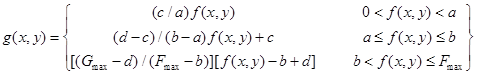close all;clear all;clc;
%分段线性灰度变换
R=imread('F:/pao1.jpg');
J=rgb2gray(R);  %将彩色图像数据R转换为灰度图像数据J
[M,N]=size(J);  %获得灰度图像J的行列数M，N
x=1;y=1;
for x=1:M
for y=1:N
if (J(x,y)<=35);  %对灰度图像J进行分段处理，处理后的结果返回给矩阵H
H(x,y)=J(x,y)*10;
elseif(J(x,y)>35&J(x,y)<=75);
H(x,y)=(10/7)*[J(x,y)-5]+50;
else(J(x,y)>75);
H(x,y)=(105/180)*[J(x,y)-75]+150;
end
end
end
figure,
subplot(121),imshow(J);
subplot(122),imshow(H);3. 非线性灰度变换当输出图像的像素点灰度值和输入图像的像素点灰度值不满足线性关系时，这种灰度变换都称为非线性灰度变换，我们以对数变换的非线性变换为例，讲解非线性灰度变换。变换公式如下：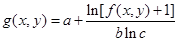close all;clear all;clc;
%基于对数变换的非线性灰度变换
R=imread('F:/pao1.jpg');
G=rgb2gray(R);  %转换成灰度图像
J=double(G);  %数据类型转换为双精度
H=(log(J+1))/10;  %进行基于常用对数的非线性灰度变换
figure,
subplot(121),imshow(G);
subplot(122),imshow(H);总结：以上三种方法给出了三种不同类型的点运算，它们的相同之处在于都能够改变图像的显示灰度，不同之处在于采用的数学方法不同。用户在需要进行图形灰度变换时，根据实际情况选择不同的运算形式，用户可参照实例设计自己的灰度变换。------想飞上天，和太阳肩并肩------
展开全文imadjust 图像处理
•   图像灰度拉伸是改变图像对比度的一种方法，通过灰度映射，将原图中某一区段中的灰度值映射到另一灰度值，从而拉伸或压缩整个图像的灰度分布范围。 2 Matlab实现 clc; clear; close all; % 对灰度图进行...
图像灰度拉伸
文章目录1 原理2 Matlab实现3 OpenCV实现4 效果
1 原理
图像灰度拉伸是改变图像对比度的一种方法，通过灰度映射，将原图中某一区段中的灰度值映射到另一灰度值，从而拉伸或压缩整个图像的灰度分布范围。
2 Matlab实现
clc;
clear;
close all;

% 对灰度图进行灰度线性变换
ori_img = imread('../images/6.jpg');
ori_img1 = rgb2gray(ori_img);
[oriHist,oriX] = imhist(ori_img1);

pt0 = [0,0];
pt1 = [100,50];
pt2 = [150,160];
pt3 = [255,200];

[width,height] = size( ori_img1);
gray1 = ori_img1;
for i=1:1:width
for j = 1:1:height
if (gray1(i,j)<pt1(1))
gray1(i,j) = pt0(2) + (gray1(i,j)-pt0(1)) * ((pt1(2)-pt0(2))/(pt1(1)-pt0(1)));
else if(gray1(i,j)>=pt1(1)&&gray1(i,j)<pt2(1))
gray1(i,j) = pt1(2) + (gray1(i,j)-pt1(1)) * ((pt2(2)-pt1(2))/(pt2(1)-pt1(1)));
else
gray1(i,j) = pt2(2) + (gray1(i,j)-pt2(1)) * ((pt3(2)-pt2(2))/(pt3(1)-pt2(1)));
end
end
end
end
[g1Hist,g1X] = imhist(gray1);
figure(1),subplot(1,2,1),imshow(ori_img1),title('原图');subplot(1,2,2),imshow(gray1),title('灰度线性拉伸');
figure(2),subplot(1,2,1),stem(oriX,oriHist),title('原图直方图');subplot(1,2,2),stem(g1X,g1Hist),title('灰度线性拉伸直方图');


3 OpenCV实现
//
int cvPointCmp(cv::Point& a, cv::Point& b)
{
return a.x < b.x;
}

//根据控制点，生成灰度拉伸所使用的查找表
void CreateLSLUT(std::vector<cv::Point>& pts, cv::Mat& lut)
{
if (pts.size() == 0)
return;
//在控制点前增加(0,0)点，末尾增加(255,255)点
std::vector<cv::Point> npts(1, cv::Point(0, 0));
npts.insert(npts.end(), pts.begin(), pts.end());
npts.push_back(cv::Point(255, 255));
//根据点的X坐标排序
std::sort(npts.begin(), npts.end(), cvPointCmp);

lut = cv::Mat(1, 256, CV_8UC1);
int nLoc = 0;
for (int i = 0; i < 256; i++)
{
for (int j = nLoc; j < npts.size()-1; j++)
{
//找出i所对应的区间的端点，左闭右开区间
if (npts[j].x <= i && npts[j+1].x > i)
{
nLoc = j;
float y = npts[j].y + 1.0*(npts[j + 1].y - npts[j].y) / (npts[j + 1].x - npts[j].x)*(i - npts[j].x);
if (y < 0)
y = 0;
if (y > 255)
y = 255;
lut.at<uchar>(i) = (uchar)y;
break;
}
}
}
}

#include "../include/baseOps.h"
#include <iostream>
#include <string>
#include "../include/opencv400/opencv2/opencv.hpp"
#include "windows.h"

int main()
{
SetCurrentDirectoryToExePath();

cv::Mat ori_img = cv::imread("../images/6.jpg");
cv::Mat gray_img;
cv::cvtColor(ori_img, gray_img, cv::COLOR_BGR2GRAY);
cv::namedWindow("灰度图");
cv::imshow("灰度图", gray_img);

cv::Mat grayHist;
calcHist1D(gray_img, grayHist);
cv::imshow("hist", grayHist);

//确定控制点
std::vector<cv::Point> pts;
pts.push_back(cv::Point(50, 100));
pts.push_back(cv::Point(100, 80));
pts.push_back(cv::Point(80, 120));
pts.push_back(cv::Point(250, 80));

//生成灰度映射表
cv::Mat lut;
CreateLSLUT(pts, lut);

//查表操作
cv::Mat res;
cv::LUT(gray_img, lut, res);

cv::Mat g1Hist;
calcHist1D(res, g1Hist);
cv::imshow("g1", res);
cv::imshow("g1Hist", g1Hist);

cv::waitKey();
return 0;
}

4 效果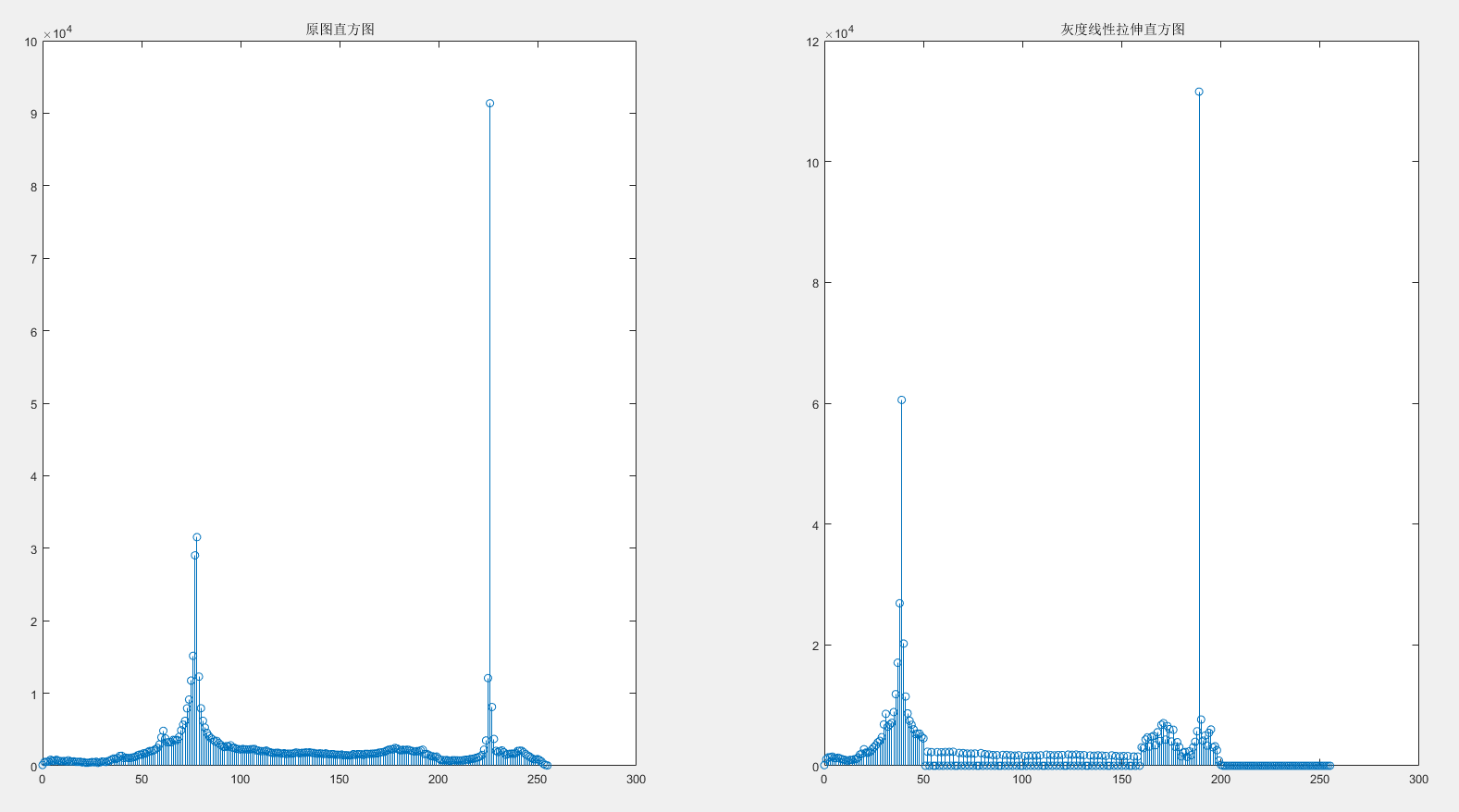展开全文• 介绍直方图均衡化，是对图像进行非线性拉伸，使得一定范围内像素的数量的大致相同。这样原来直方图中的封顶部分对比度得到了增强，而两侧波谷的对比度降低，输出的直方图是一个较为平坦的分段直方图。具体来讲可以...
前言这是OpenCV图像处理专栏的第七篇文章，主要为大家介绍一下直方图均衡化算法的原理以及提供一个我的C++代码实现。介绍直方图均衡化，是对图像进行非线性拉伸，使得一定范围内像素值的数量的大致相同。这样原来直方图中的封顶部分对比度得到了增强，而两侧波谷的对比度降低，输出的直方图是一个较为平坦的分段直方图。具体来讲可以表现为下面这个图：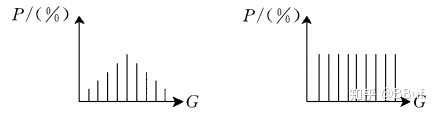通过这种方法可以按照需要对图像的亮度进行调整，并且，这种方法是可逆的，也就是说知道了均衡化函数，也可以恢复原始的直方图。算法原理设变量代表图像中像素灰度级。对灰度级进行归一化处理，即，其中表示黑，表示白。对于一幅给定的图片来说，每个像素在的灰度级是随机的，用概率密度来表示图像灰度级的分布。为了有利于数字图像处理，引入离散形式。在离散形式下，用代表离散灰度级，用代表，并且下式子成立：，其中。式子中代表图像中出现这种灰度的像素个数，是图像的总像素个数，图像进行直方图均衡化的函数表达式为:，式子中，为灰度级数(RGB图像为255)。相应的反变换为代码实现//直方图均衡化
Mat Histogramequalization(Mat src) {
int R = {0};
int G = {0};
int B = {0};
int rows = src.rows;
int cols = src.cols;
int sum = rows * cols;
//统计直方图的RGB分布
for (int i = 0; i < rows; i++) {
for (int j = 0; j < cols; j++) {
B[src.at<Vec3b>(i, j)]++;
G[src.at<Vec3b>(i, j)]++;
R[src.at<Vec3b>(i, j)]++;
}
}
//构建直方图的累计分布方程，用于直方图均衡化
double val = {0};
for (int i = 0; i < 256; i++) {
val += B[i];
val += G[i];
val += R[i];
B[i] = val * 255 / sum;
G[i] = val * 255 / sum;
R[i] = val * 255 / sum;
}
//归一化直方图
Mat dst(rows, cols, CV_8UC3);
for(int i = 0; i < rows; i++){
for(int j = 0; j < cols; j++){
dst.at<Vec3b>(i, j) = B[src.at<Vec3b>(i, j)];
dst.at<Vec3b>(i, j) = G[src.at<Vec3b>(i, j)];
dst.at<Vec3b>(i, j) = R[src.at<Vec3b>(i, j)];
}
}
return dst;
}效果原图直方图均衡化后的图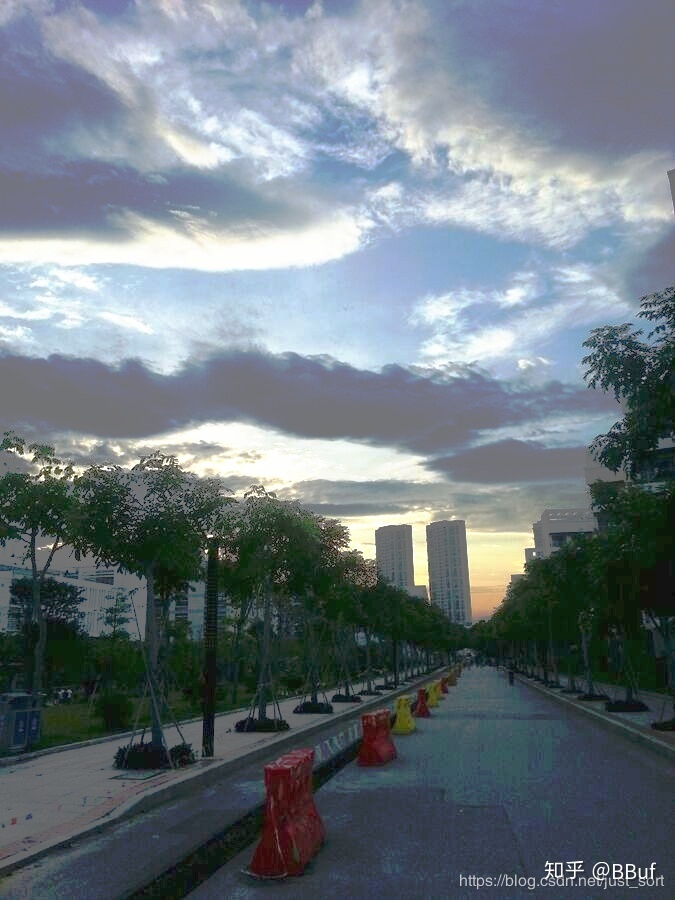后记本文为大家介绍了直方图均衡化算法，以及它的简单代码实现，希望可以帮助到你。欢迎关注我的微信公众号GiantPandaCV，期待和你一起交流机器学习，深度学习，图像算法，优化技术，比赛及日常生活等。展开全文• MATLAB中，通过函数imadjust是一个计算机函数,该函数用于调节灰度图像的亮度或彩色图像的颜色矩阵，该函数调用格式如下： J=imadjust( I ) 对图像I进行灰度...将灰度图像 I 中的亮度映射到 J 中的新，使得图...
• 一、将一幅图像转化为二图像,可以...BW = im2bw(RGB, level)%将RGB彩色图像转换为二图像，转换过程中先转化为灰度图像，再转换为二图像 二图像：取值只有0和1的逻辑数组（logical型的数组）。如果是一个
• Automatic Contrast Adjustment的目的是将图像的灰度范围从[alow,ahigh][a_{low}, a_{high}][alow​,ahigh​]线性拉伸/压缩到[amin,amax][a_{min}, a_{max}][amin​,amax​]。 计算分为两步： 找到图像中像素的最值...
• （3）, 根据线性公式，求拉伸灰度值，如下图1. matlab代码：%题目： 对比度拉伸 %意义：所期望观察的对象因对比度不足而不够清晰，需进行对比度拉伸。 %灰度值的分段线性映射 %已知条件：原图像的目标景物灰度...
• 【功能】使用去相关拉伸增强图像相关区域的颜色。 【语法介绍】 ...·S=decorrstretch(I)对图像I进行去相关拉伸。...·S=decorrstretch(I,_tol’，TOL)对图像I进行去相关...TOL为二元向量时，表示所增强的灰度值较小
• 对数变换可以拉伸范围较窄的低灰度值，同时压缩范围较宽的高灰度值。可以用来扩展图像中的暗像素值，同时压缩亮像素值。简而言之是对图像中低灰度细节进行增强其中 为常数， , 可以使函数左移一个单位，得到的s均...
• 灰度线性变换和灰度拉伸是对像素灰度值的变换操作，直方图是对像素灰度值的统计，直方图均衡是对灰度值分布的变换。1.灰度线性变换(1)线性变换函数原图向灰度值为g。通过线性函数f(x)=kx+b转换为f(g)得到灰度的线性...
• 实验当中总要可视化自己的数据，比方说标签是33类，你要可视化，单纯的灰度值拉伸不明显，如果是11类那会明显很多，但是类别越多，边界线越模糊，所以最好还是上彩色图像。但是上色程序里面的颜色值又不能随机化，...
• VC跟Matlab混合编程VC与Matlab案例-VC_MATLAB案例.rar 1.256色转灰度图 2.对比度拉伸 3.二化变换 4.反色 5.灰度均衡 6.亮度增减 7.取对数 8.取指数 9.图像镜像 10.图像平移 11.阈值变换 12.直方图...
• 图像点处理是图像处理系列的基础，主要用于让我们熟悉Matlab图像处理的编程环境。灰度线性变换和灰度拉伸是对像素灰度值的变换操作，直方图是对像素灰度值的统计，直方图均衡是对灰度值分布的变换图像处理 线性变换 直方图均衡化
• 而当使用imshow(A, [])后则matlab会自动拉伸灰度值，将1,2,4变化到0,85,255.这样图像看起来对比度明显，肉眼也能分辨。 具体计算方式为： ((原始的灰度值 - min(A))/(max(A)-min(A)))*255 = 新的灰度值 ((1-1...
• 灰度线性变换和灰度拉伸是对像素灰度值的变换操作，直方图是对像素灰度值的统计，直方图均衡是对灰度值分布的变换。 1.灰度线性变换 （1）线性变换函数 原图向灰度值为g，通过线性函数f(x)=kx+b转换为f(g)...
• 为了后续处理方便，在多类目标的掩膜图像中，灰度值经常设置为0,1,2,3…，分割的结果不经过灰度拉伸根本看不出来长什么样子，将灰度值拉伸后又害怕后续处理麻烦，因此便想出来，直接将图像存储成彩色索引图像就行。...计算机视觉
• 点运算又称为对比度增强、对比度拉伸或灰度变换，是一种通过图像中的每一个像素值(即像素点上的灰度值)进行运算的图像处理方式。它将输入图像映射为输出图像，输出图像每个像素点的灰度值仅由对应的输入像素点的灰度...
• 对比度拉升采用了线性函数对图像的灰度值进行变换，例如：空间滤波：线性滤波(拉普拉斯边缘、均值、高斯等)非线性滤波(中值等)频域：频域滤波低通滤波器(图像模糊、平滑)高通滤波高频强调滤波均值滤波去除椒盐噪声：...
• 为便于实现，可以用查找表（look-up table）的方式存储，即：原始的灰度作为查找表的索引，表中的内容是新的灰度值。 其次，直方图均衡化是图像增强的一种基本方法，可提高图像的对比度，即：将较窄的图像灰度...
• ## Matlab 直方图均衡化

万次阅读 多人点赞 2016-11-16 09:40:37
直方图均衡化介绍直方图均衡化是图像增强的一种基本方法，可提高图像的对比度，即：将较窄的图像灰度范围以一定规则拉伸至较大（整个灰度级范围内）的范围。 目的是在得到在整个灰度级范围内具有均匀分布的图像。 ...
• 它将输入图像映射为输出图像，输出图像每个像素点的灰度值仅由对应的输入像素点的灰度值决定，运算结果不会改变图像内像素点之间的空间关系。简而言之，点运算仅仅改变了图像像素点的灰度值。 其运算的数学关系式为...
• 直方图：把原始图像的灰度直方图从比较集中的某个灰度区间变成在...直方图均衡化的基本思想是把原始图的直方图变换为均匀分布的形式，这样就增加了象素灰度值的动态范围从而可达到增强图像整体对比度的效果。 图像的
• 对直方图进行均衡化处理的源代码： 把原始图像的灰度直方图从比较集中的...对图像进行非线性拉伸，重新分配图像像素，使一定灰度范围内的像素数量大致相同。把给定图像的直方图分布改变成“均匀”分布直方图分布。
• imshow(I,[low,high])：I中灰度值低于low的会显示为黑色，高于high的会显示为白色，介于low和high之间的会被线性拉伸到0-255显示； imshow(I,[])：函数会将图像矩阵中min(I(:))=low，max(I(:))=high；从而达到灰度...
• 点运算（对比度增强、对比度拉伸或灰度变换），是一种通过图像中的每一个像素值（即像素点上的灰度值）进行运算的图像处理方式。 线性灰度变换 分段线性灰度变换 非线性灰度变换 图像代数运算 图像逻辑运算 图像的...
• 图像点运算点运算又称为对比度增强，对比度拉伸灰度拉伸，是通过图像中的每一个像素进行运算的图像处理方式，运算不会改变图像像素点之间的空间关系。MATLAB中通过 imadjust()进行线性灰度变...# matlab灰度值拉伸matlab 订阅